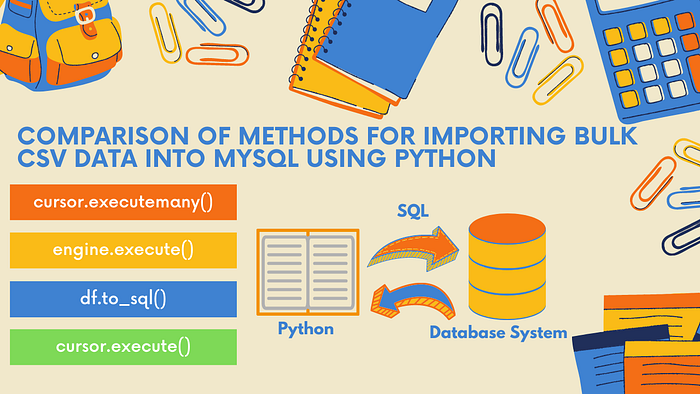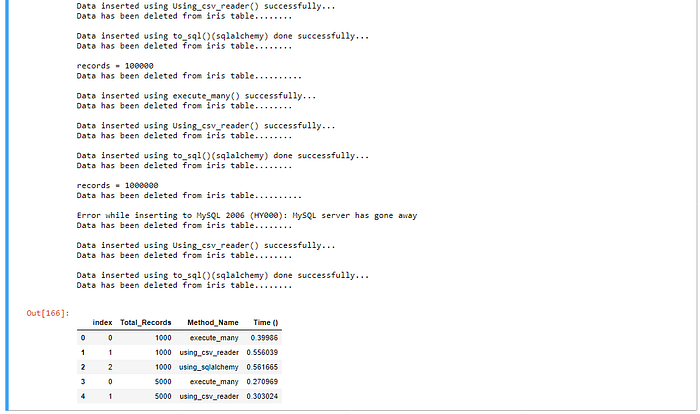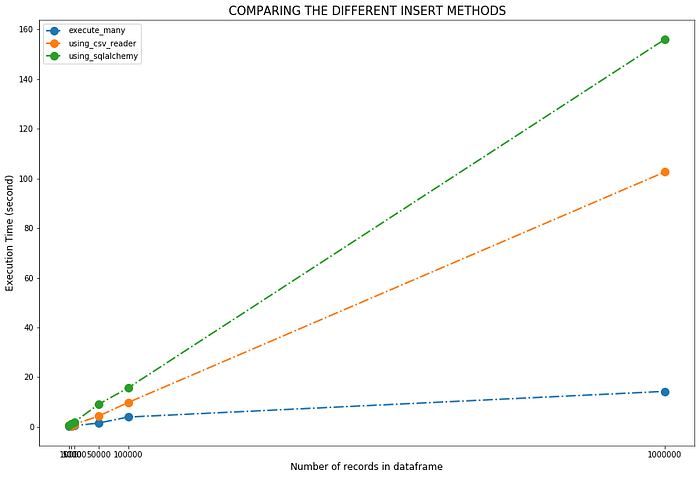# Importing Bulk CSV Data Into MySQL Using Python

## Find the Fastest Way to Import CSV Data Into MySQL Database Using Python### 1. Overview

The main objective of this tutorial is to find the best method to import bulk CSV data into MySQL.

### 2. Prerequisites

sqlalchemy : To install sqlalchemy use the command:

``````pip install sqlalchemy
``````

### 3. Prepare or Identify Your Data

To begin, prepare or identify the CSV file that you'd like to import to MySQL database. For example, we loaded iris data from GitHub.

#### 3.1 Import Libraries

``````import os
import sys
import pandas as pd
import numpy as np
from sqlalchemy import create_engine
from sqlalchemy.exc import SQLAlchemyError
import seaborn as sns
import matplotlib.pyplot as plt
import mysql.connector as msql
from mysql.connector import Error
import csv
``````

#### 3.2 Extract the Data

``````# Loading data from github
df = irisData
``````

#### 3.3 Create a Table in MySQL Database

To connect from Python to a MySQL database, we use sqlalchemy and mysql.connector:

Specify the connection parameters

``````# Note: please change your database, username & password as per your own values
conn_params_dic = {
"host"      : "localhost",
"database"  : "irisdb",
"user"      : "root",
}
``````

Define function to establish connection mysql.connector

``````# Define a connect function for MySQL database server
def connect(conn_params_dic):
conn = None
try:
print('Connecting to the MySQL...........')
conn = msql.connect(**conn_params_dic)
print("Connection successfully..................")

except Error as err:
print("Error while connecting to MySQL", err)
# set the connection to 'None' in case of error
conn = None
return conn
``````

Define function to establish connection using Alchemy

``````# Using alchemy method
connect_alchemy = "mysql+pymysql://%s:%s@%s/%s" % (
conn_params_dic['user'],
conn_params_dic['host'],
conn_params_dic['database']
)

def using_alchemy():
engine = None
try:
print('Connecting to the MySQL...........')
engine = create_engine(connect_alchemy)
print("Connection successfully..................")
except SQLAlchemyError as e:
err=str(e.__dic__['orig'])
print("Error while connecting to MySQL", err)
# set the connection to 'None' in case of error
engine = None
return engine
``````

Define a function to create table

``````def create_table(engine):
try:
# Dropping table iris if exists
engine.execute("DROP TABLE IF EXISTS iris;")
sql = '''CREATE TABLE iris(
sepal_length DECIMAL(2,1) NOT NULL,
sepal_width DECIMAL(2,1) NOT NULL,
petal_length DECIMAL(2,1) NOT NULL,
petal_width DECIMAL(2,1),
species CHAR(11) NOT NULL
)'''
# Creating a table
engine.execute(sql);
print("iris table is created successfully...............")
except Error as err:
print("Error while connecting to MySQL", err)
# set the connection to 'None' in case of error
conn = None
``````

### 4. Running Time

For measuring execution time of each method, we used timeit.

``````# Example
def run_method(n):
for i in range(n):
3 ** nfrom timeit import default_timer as timer
start_time = timer()
run_method(10000)
end_time = timer()
elapsed = end_time-start_time
print('function took {:.3f} ms'.format((elapsed)*1000.0))
``````

### 5. Methods

#### 5.1. Using one by one inserts

To establish a baseline we start with the easiest methodology, insert records one by one:

``````def single_inserts(engine, df, table):
for i in df.index:
cols  = ','.join(list(df.columns))
vals  = [df.at[i,col] for col in list(df.columns)]
query = "INSERT INTO %s(%s) VALUES(%s,%s,%s,%s,'%s')" % (table, cols, vals, vals, vals,vals,vals)
engine.execute(query)
print("single_inserts() done")
``````

#### 5.2. Using execute_many()

The mysql documentation : using executemany

Execute a database operation (query or command) against all parameter tuples or mappings found in the sequence vars_list.

``````# Define function using cursor.executemany() to insert the dataframe
def execute_many(conn, datafrm, table):

# Creating a list of tupples from the dataframe values
tpls = [tuple(x) for x in datafrm.to_numpy()]

# dataframe columns with Comma-separated
cols = ','.join(list(datafrm.columns))

# SQL query to execute
sql = "INSERT INTO %s(%s) VALUES(%%s,%%s,%%s,%%s,%%s)" % (table, cols)
cursor = conn.cursor()
try:
cursor.executemany(sql, tpls)
conn.commit()
print("Data inserted using execute_many() successfully...")
except Error as e:
print("Error while inserting to MySQL", e)
cursor.close()
``````

``````def using_csv_reader(engine, datafrm, table_name):

try:
# Here, change your own path to dump the temp file
datafrm.to_csv('./Learn Python Data Access/iris_bulk.csv', index=False)
# dataframe columns with Comma-separated
cols = ','.join(list(datafrm.columns))
# SQL query to execute
sql = "INSERT INTO %s(%s) VALUES(%%s,%%s,%%s,%%s,%%s)" % (table_name, cols)
sql = sql.format(table_name)

with open('./Learn Python Data Access/iris_bulk.csv') as fh:
#sub_sample = [next(fh) for x in range(records)]
engine.execute(sql, data)
except Error as err:
print("Error while inserting to MySQL", e)
``````

#### 5.4. Using to_sql() (sqlalchemy)

``````def using_sqlalchemy(engine,datafrm,table):
try:
datafrm.to_sql(table, con=engine, index=False, if_exists='append',chunksize = 1000)
print("Data inserted using to_sql()(sqlalchemy) done successfully...")
except SQLAlchemyError as e:
error = str(e.__dic__['orig'])
print("Error while inserting to MySQL", error)
``````

### 6. Results

``````conn = connect(conn_params_dic)
engine = using_alchemy()
create_table(engine)
``````

Define a function to compare the performance of each method

Here, we are running only three methods execute_many, using_csv_reader, and using_sqlalchemy because we know already single inserts will take more time compare to other three. So, if you want to check performance time for single inserts also then you can uncomment below code.

``````#-----------------------------------------
# COMPARE THE PERFORMANCE OF EACH METHOD
#-----------------------------------------
def compare_methods_to_insert_bulk_data(conn,engine,df):
# Delete records from iris table
engine.execute("delete from iris where true;")
print("Data has been deleted from iris table..........")
print("")

# Including single_inserts method
#methods = [single_inserts, execute_many, using_csv_reader, using_sqlalchemy]
# Excluding single_inserts method

df_performance = pd.DataFrame(index=range(len(methods)), columns=['Total_Records','Method_Name','Time ()'])k = 0
for method in methods:
start_time = timer()
if method==execute_many:
method(conn, df, 'iris')
else:
method(engine, df, 'iris')
end_time = timer()

df_performance.at[k,'Total_Records'] = len(df.index)
df_performance.at[k,'Method_Name'] = method.__name__
df_performance.at[k,'Time ()'] = end_time-start_time

# Delete records for the previous method and prepare test for the next method
engine.execute("delete from iris where true;")
print("Data has been deleted from iris table........")
print("")
k = k + 1

return df_performance
``````

Compare the performance of each method for 1000, 5000,10000, 50000,100000,1000000 records.

``````df = irisData
# Repeating our dataframe 6667 times to get a large test dataframe
bulk_df = pd.concat([df]*6667, ignore_index=True)
print(len(bulk_df.index))df_performance_list = []
for records in [1000,5000,10000,50000,100000,1000000]:
print("records = %s" % records)
df_cutoff = bulk_df[0:records]
df_performance = compare_methods_to_insert_bulk_data(conn,engine,df_cutoff)
df_performance_list.append(df_performance)method_performances = pd.concat(df_performance_list, axis=0).reset_index()
``````Visualize the performance of each method

``````fig, ax = plt.subplots(figsize=(15,10))
for method in method_performances['Method_Name'].unique():
subset = method_performances[method_performances['Method_Name'] == method]
ax.plot(subset['Total_Records'], subset['Time ()'], 'o-.', label=method, linewidth=2, markersize=10)plt.xticks([1000, 5000,10000, 50000,100000,1000000])
plt.xlabel('Number of records in dataframe',fontsize=12)
plt.ylabel('Execution Time (second)',fontsize=12)
plt.title("COMPARING THE DIFFERENT INSERT METHODS", fontsize=15)
plt.legend()
plt.savefig(".../Learn Python Data Access/all_methods.png", dpi=600)
plt.show()
````````````# Closing the cursor & connection
cursor.close()
conn.close()
``````

### 7. Conclusion

Now, the main question is what should we use? the answer is it depends. Each method has its own advantages and disadvantages, and is suited for different circumstances. Here, we used very simple data with only five variables. Data can be complex , and system configuration can be different . As per our example, the fastest method is to use execute_many() however, using csv_reader() is also fine method to import the data.